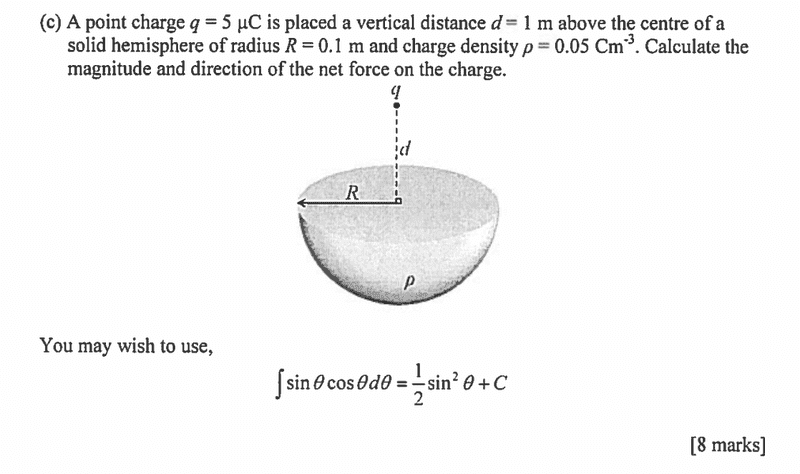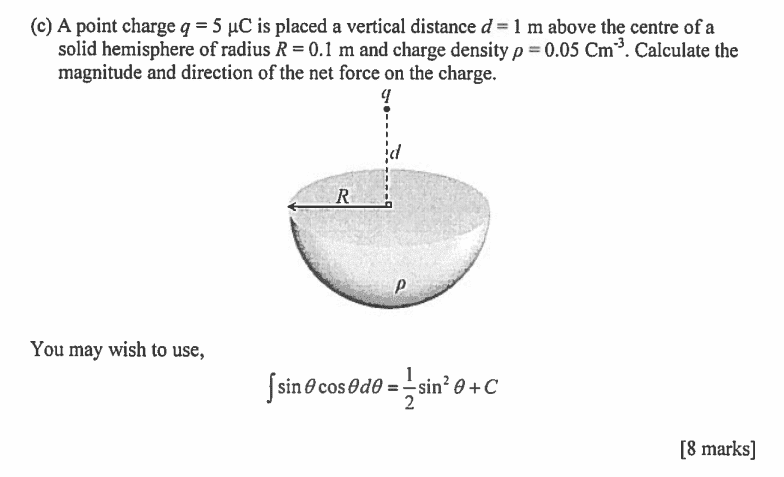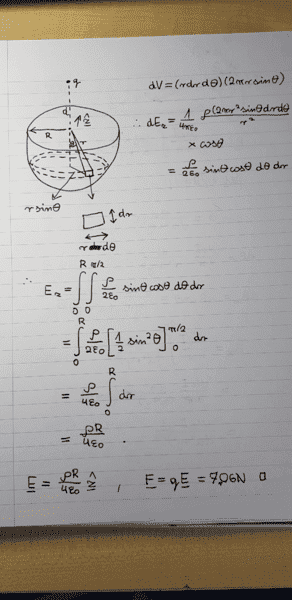# Confusion about the Lorentz force

Silicon-Based
Member advised to use the formatting template for all homework help requestsWe can find the force by finding the E-field on the charge first, then applying Lorentz force formula. However, it isn't obvious to me at all how to find the E-field. If the charge were on top of the hemisphere I would be using spherical coordinates, but here I don't know which coordinate system to even use.

#### Attachments

Homework Helper
What happened to the template ?
You'll need a few equations to get started.
If I were you, I'd use spherical coordinates with the center of the hemisphere as center and the hemisphere in ##\theta\le 0##

Silicon-Based
If I were you, I'd use spherical coordinates with the center of the hemisphere as center and the hemisphere in ##\theta\le 0##

Could you elaborate on that? How could I do that to then find the E-field at the point where the charge is?

Homework Helper
Actually, you should be the one to do that ! You want to set up an integral of something like ## {dq \over r^2} = \rho {dV\over r^2}##
Since we chose the coordinate system so nifty, there is only one component of interest. What is ##dV## in terms of spherical coords ?

Silicon-Based
Actually, you should be the one to do that ! You want to set up an integral of something like ## {dq \over r^2} = \rho {dV\over r^2}##
Since we chose the coordinate system so nifty, there is only one component of interest. What is ##dV## in terms of spherical coords ?
I believe it should be ##dV=r^2 \sin{\theta}d\phi d\theta dr##

Homework Helper
Dearly Missed
I believe it should be ##dV=r^2 \sin{\theta}d\phi d\theta dr##
Keep going.

Silicon-Based
I still don't see where this is going. I integrated for ##Q## and got ##Q=2\pi R \rho r^2##, if that's in any way helpful. Regardless of weather this is right or not, this would give me the total charge of the hemisphere, but I don't see how I'm supposed to use it to find the force; we can't treat the hemisphere as a point particle.

Homework Helper
Dearly Missed
I still don't see where this is going. I integrated for ##Q## and got ##Q=2\pi R \rho r^2##, if that's in any way helpful. Regardless of weather this is right or not, this would give me the total charge of the hemisphere, but I don't see how I'm supposed to use it to find the force; we can't treat the hemisphere as a point particle.

I suggest you go back to the start, and forget about spherical coordinates until you have successfully set up the correct integration. Then, and only then, can you decide if spherical coordinates are helpful.

So, you have a lot of little charges ##dQ = \rho dV## at positions ##(x,y,z)## in the hemisphere. The ##z-##component of force on the test charge due to charge ##dQ## at ##(x,y,z)## is
$$dF_z = k q \rho \, \frac{dx \, dy \, dz}{x^2 + y^2 + (d-z)^2}.$$
This is to be integrated over the 3-dimensinal region ##\{ 0 \geq z \geq -\sqrt{R^2 - x^2 - y^2} \}##.

WARNING: the above is not quite correct---I made an error. I will leave it up to you to figure out what changes are needed.

Last edited:
Homework Helper
Here Ray has already leaked that the net force on q is in the z direction. No big secret: symmetry in ##\phi## (i.e. around the ##z##-axis) does that for you.

Silicon-Based
I suggest you go back to the start, and forget about spherical coordinates until you have successfully set up the correct integration. Then, and only then, can you decide if spherical coordinates are helpful.

So, you have a lot of little charges ##dQ = \rho dV## at positions ##(x,y,z)## in the hemisphere. The ##z-##component of force on the test charge due to charge ##dQ## at ##(x,y,z)## is
$$dF_z = k q \rho \, \frac{dx \, dy \, dz}{x^2 + y^2 + (d-z)^2}.$$
This is to be integrated over the 3-dimensinal region ##\{ 0 \geq z \geq -\sqrt{R^2 - x^2 - y^2} \}##.

To be frank, I've been only exposed to derivatives of polar coordinates. These are the ones that come up in E&M most of the time. So it's actually easier for me to set up the integration with spherical coordinates in mind. Moreover, it's obvious some offshoot of those need to be used, given the hint below the question.

The integral I evaluated was:
$$\int\limits_{0}^{R}\int\limits_{0}^{\pi/2}\int\limits_{0}^{2\pi} \rho \sin{\theta} d\phi d\theta dr$$

Homework Helper
Dearly Missed
Here Ray has already leaked that the net force on q is in the z direction. No big secret: symmetry in ##\phi## (i.e. around the ##z##-axis) does that for you.

Not really; I just talked about the ##z##-component of the force.

However, what I wrote is not quite correct! The OP can figure out how to fix it.

Homework Helper
I miss where the ##r^2## went: you gave the correct ##dV=r^2 \sin{\theta}d\phi d\theta dr## so that Q comes out as a much more sensible ##{2\over 3} \pi \rho R^3##

Last edited:
Homework Helper
Not really; I just talked about the zzz-component of the force.
I stand corrected.Homework Helper
To be frank, I've been only exposed to some derivatives of polar coordinates. These are the ones that come up in E&M most of the time. So it's actually easier for me to set up the integration with spherical coordinates in mind. Moreover, it's obvious some offshoot of those need to be used, given the hint below the question.

Sketch how a small volume of charge at ##r,\theta## (e.g. in the x,z plane) generates an ##\vec E## at the position of q.

Last edited:
Homework Helper
Dearly Missed
To be frank, I've been only exposed to derivatives of polar coordinates. These are the ones that come up in E&M most of the time. So it's actually easier for me to set up the integration with spherical coordinates in mind. Moreover, it's obvious some offshoot of those need to be used, given the hint below the question.

The integral I evaluated was:
$$\int\limits_{0}^{R}\int\limits_{0}^{\pi/2}\int\limits_{0}^{2\pi} \rho \sin{\theta} d\phi d\theta dr$$

I don't see how you got this; you need more explanation.

Silicon-Based
I miss where the ##r^2## went: you gave the correct ##dV=r^2 \sin{\theta}d\phi d\theta dr## so that Q comes out as a much more sensible ##{2\over 3} \pi R^3##

I must have misinterpreted your earlier post:
You want to set up an integral of something like ## {dq \over r^2} = \rho {dV\over r^2}##

If I were to keep ##r^2## in the integrand, I'd get the right answer.

Sketch how a small volume of charge at ##r,\theta## (e.g. in the x,z plane) generates an ##\vec E|## at the position of q.

Well, if ##r## is the distance from the charge, and ##\theta## the angle between ##r## and the ##z##-axis, the E-field due to a small charge is given by ##dE=k\frac{dQ}{r^2}##, so to find the E-field in the desired direction, we have ##dE_z=k\frac{dQ}{r^2}\cos{\theta}##

Silicon-Based
I don't see how you got this; you need more explanation.
As before, in response to BvU. If I were to keep ##r^2## in the integrand, I would get the right answer.

Homework Helper
Dearly Missed
As before, in response to BvU. If I were to keep ##r^2## in the integrand, I would get the right answer.

Doesn't ##r## change when you change the location ##(x,y,z)## of the source charge ##\rho \, dV?## Because of the way you wrote the force expression, I thought that ##r## was the distance from the test charge ##q## at ##(0,0, d)## and the source charge at ##(x,y,z)##. Of course, maybe you want ##r## to be the distance from the origin to ##(x,y,z),## but in that case you need a new distance ##r' = \sqrt{x^2 + y^2 + (d-z)^2}## in the force expression.

Or, are you claiming that the field at ##(0,0,d)## is the same as that of a point charge ##Q = \rho V##, located at the origin? I have serious doubts about that.

It is true that the field due to a uniformly charged sphere (or spherical shell) is the same as for a point charge located at the center, but a hemisphere is not a sphere.

https://physics.stackexchange.com/questions/363196/charged-hemisphere

Last edited:
Homework Helper
I must have misinterpreted your earlier post:
Yes, that was for the ##\vec E## already, (with a different ##r## !)

Make the sketch. You'll need to distinguish several ##r##.

Silicon-Based
Thread moved from the technical forums, so no Homework Template is shown
The following is a past exam question on electrostatics:And here is my (correct) solution:I understand the derivation of the E-field at the centre of the flat surface of the hemisphere, but I don't see the justification for using it in the Lorentz force formula. Is one not supposed to use the Lorentz formula for the charge and the E field at the same point in space, not a distance d away? The resulting formula has no spatial variable, which would imply that placing the charge on the same axis but at a large distance away from the hemisphere would result in the exact same force (while it obviously should decrease with distance).

#### Attachments

You haven't set up the integral correctly. As you said, you want to calculate the E-field where the charge is. In your integral, you are summing up the contribution of each infinitesimal packet of charge at location (r, theta, phi) to the field at the point where the charge is. To do this, the 1/r^2 term in your integrand is the distance from the charge to the point in the charged sphere. So it needs to contain the distance d. What is the distance from the point charge to the point in the charged sphere in terms of d, r, theta and phi? Also, you need to sum up each component of E separately, or use symmetry arguments to explain why some components of E might be zero.

Silicon-Based
My solution is correct. I should not find the E-field at the point where the charge is, that would be far beyond my abilities. This solution is pretty much the model solution for that question (I dicussed it wih the examiner himself), but now the examiner refuses to respond to my email to clarify my concern in question.

Mentor
I agree with phyzguy's comments. You found the field at the center of the hemisphere, not at a distance 'd' above that center.

Silicon-Based
So it's my electromagnetism course that is messed up? Great... supposedly the best university in Europe, and it can't get a freshman EM course right.

My solution is correct. I should not find the E-field at the point where the charge is, that would be far beyond my abilities. This solution is pretty much the model solution for that question (I dicussed it wih the examiner himself), but now the examiner refuses to respond to my email to clarify my concern in question.

So it's my electromagnetism course that is messed up? Great... supposedly the best university in Europe, and it can't get a freshman EM course right.

Why would it be "beyond you capabilities"? You've already set up an integral and evaluated it. You just need to make a modification. If you're taking an EM course at " the best university in Europe", you should be able to calculate the distance from the point d above the center to a point (r, theta phi). It is simple geometry. If you can't do that, I would suggest that it is not your course which is "messed up".

Silicon-Based
Why would it be "beyond you capabilities"? You've already set up an integral and evaluated it. You just need to make a modification. If you're taking an EM course at " the best university in Europe", you should be able to calculate the distance from the point d above the center to a point (r, theta phi). It is simple geometry. If you can't do that, I would suggest that it is not your course which is "messed up".

Look, this is THE MODEL SOLUTION that I cosulted with the examiner who made up this question himself. I'm asking for a clarification of one of the steps.

As for the approach you suggest, this is far from simple geometry. I tried to solve it that way for at least 2 weeks, consulting various forums and people, until I had to get in contact with the examiner himself. I'm not supposed to be using anything beyond simple cathesian coordinates, or spherical/cyllindrical coordinates.

Mentor
Look, this is THE MODEL SOLUTION that I cosulted with the examiner who made up this question himself.
By your own questions, I think you realize that this solution cannot be correct, despite what the examiner says. Is he the professor for the course or a teaching assistant? When you consulted with him, did you ask why the answer did not depend on the distance "d"?

Silicon-Based
By your own questions, I think you realize that this solution cannot be correct, despite what the examiner says. Is he the professor for the course or a teaching assistant? When you consulted with him, did you ask why the answer did not depend on the distance "d"?

He's the lecturer of the course. I tried to inquire about that exact detail at that time but I was still partly in the process of digesting the integration. I have hence tried to get in touch with the examiner again (by email, his lecture course ended) but he did not reply to me yet.

Silicon-Based
Update: the examiner has just responded to me, and indeed, he made a mistake; there should be no spatial separation between the charge and the centre of the hemisphere.

Mentor
Update: the examiner has just responded to me, and indeed, he made a mistake; there should be no spatial separation between the charge and the centre of the hemisphere.
Very good. Sanity has been restored. (I'm glad he owned up!)